# Poisson equation, numerical methods

Methods replacing the original boundary value problem for the Poisson equation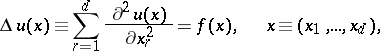(1)

by a system oflinear algebraic equations(2)

with a solutionwhich makes it possible to construct a certain approximationto the solution of the original problem as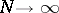.

One defines such extremely important concepts as the error of the numerical method and bounds for the error (the accuracy) depending on the way one compares the solutions of the original problem (1) and the discrete problem (2). The algebraic properties of the system (2) (discrete analogues of boundary value problems) connected with the stability of solutions (the well-posedness of the discrete problems), the possibility of finding exact or approximate solutions of (2) by some direct or iterative method when carrying out the corresponding computation and corresponding demands on the computer memory (see Minimization of the labour of calculation) give other characteristics of the numerical methods.

Numerical solutions of boundary value problems for the Poisson equation are important not only because these problems often arise in diverse branches of science and technology, but because they frequently are a means for solving more general boundary value problems for both equations and systems of equations of elliptic type as well as for various non-stationary systems. The basic numerical methods for solving the boundary value problems under discussion are projection methods and difference methods (see ).

## Projection methods.

These encompass a number of methods: variational, least squares, Galerkin, projection-difference, projection-grid, and finite elements methods. In all of these one characteristically reduces the original boundary value problem to an operator equation(3)

(the operatoracts, for example, from a Hilbert spaceinto) with subsequent choice of finite-dimensional subspacesand(): the problem (3) itself is replaced in these methods by the problem of finding asuch that for any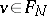,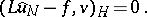Then, given bases in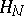and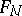, the system (2) is a system for the coefficients in the expansion of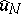with respect to the basis ofand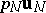can be taken as the functionitself; it is natural to define the error in the method as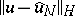. In the most important casesis a certain subspace of the Sobolev space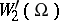,and, if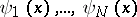is a basis of, then the system (2) takes the form(4)The error in the method is estimated by the distance infrom the solution of the original problem to the subspace(see , ). In modern variants of projection methods the subspacestend to be chosen so that the functionshave local supports and in each equation (4) only a finite number of coefficients are non-zero. Methods of this type are also called projection-grid methods (projection-difference, variational-difference, finite element methods) (see , , ). The great merit of these methods is that they can be applied when the domainof the boundary value problem under consideration is geometrically fairly complicated. Methods which are also close to projection methods but relatively rarely applied are the collocation method, spectral methods and boundary element methods (see ).

## Difference (finite-difference) methods.

These use a certain grid domaincontainingnodes of a grid to approximate the original domainand usually lead to a system (2) by approximating the Poisson equation and the corresponding boundary conditions by their difference (grid) analogues, using only the values of the function at the chosen nodes (see ). The error of the method is usually obtained by comparing the vector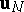and the vector obtained by restricting the required solution to the set of nodes under consideration. The well-posedness and approximation can be studied for various choices of norms; it is, in particular, possible to use the maximum principle; convergence is obtained as a corollary of well-posedness and approximation (see ).

Systems (2) can be derived both by basing them on certain discrete analogues of the corresponding variational problems and by basing them on an approximation to certain integral relations (see , , , ); such approaches bring these variants of difference methods somewhat closer to projection-difference methods.

The most intensively studied methods for solving systems of grid equations (2) are the simplest difference analogues on a parallelepiped grid (see , ). In the case of two variables and when the domainin the plane is rectangular, direct methods are often applied for a number of boundary conditions; these direct methods enable one to find the solution of (2) at the cost of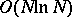arithmetic operations. Such a method is that of separation of variables, which uses the discrete Fourier transform and the reduction method (cf. Separation of variables, method of); methods are also known with a boundon the numbers of operations (see ). Whenand one may use separation of variables (in this caseis a parallelepiped), the solution of (2) can be found with accuracyat the cost of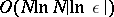operations by an iterative alternating-directions method (see , ); iterative methods with factorizable operators (methods of successive overrelaxation with symmetrization, incomplete matrix factorization, alternating-triangular methods) enable one to find the solution of (2) with accuracyat a cost ofoperations (see , ) in fairly general situations.

In the case of simply-connected and multiply-connected domainsin the plane which are composed of a finite number of rectangles, the solution of the system (2) with accuracycan be found at the cost ofoperations by cuttingup into rectangles (see , ). Similar asymptotic solutions for discrete analogues of the Dirichlet problem can be obtained for certain domains using the methods of capacity and of fictitious unknowns (see , ). For a number of systems (2) which are projection-difference analogues of the original problem, computation lengths of the orderand sometimes also(whenis of order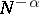,) can be achieved by iterative methods using the spectral equivalence of operators (see , ). In a number of cases the use of a sequence of grids enables one to obtain methods which give the solution to (2) with an accuracy of order,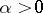, and with asymptotically-minimal computational work (the number of operations is) (see for example , , ).